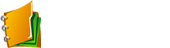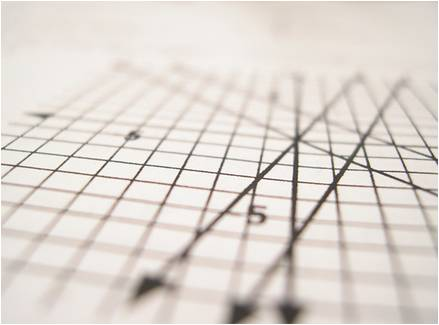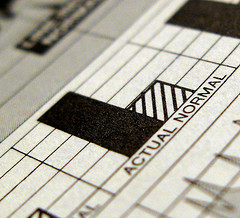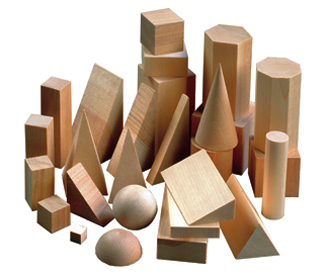﻿ Math 11 Assignments
 FAIL (the browser should render some flash content, not this). "Thank you for these assignments. I learn a lot more from doing them than the textbooks we use at school. " Highschool student, Vancouver School DistrictMath 11 Ch 1: Sequences and Series

Assignments

SOL

1.3

Practice Test and QuizMath 11 Ch 2: Trigonometry

Assignments

SOL

2a
2.1

2.1b
2.2

Practice Test and QuizMath 11 Ch 3 & 4: Quadratic Functions

Assignments

SOL

A2B

A3
A3

A10

C3R

Practice Test and Quiz

•  Quiz 4a Factoring & Solving Trinomials
•  Quiz Lesson 1 to Lesson 5
•  Chapter 3 Practice Test A
•  Chapter 3 Practice Test B
•  Chapter 3/4 Practice Test C
•  Chapter 3 Review
•  Desmos Domain and RangeAssignments

SOL

Practice Test and Quiz

Chapters 3 and 4 are combined together as one chapter. Students are encouraged to take an algebraic approach with the graphical approach as a secondary source.Math 11Ch 5: Exponent Rules with Radicals Expressions and Equations

Assignments

SOL

Practice Test and Quiz

•  Quiz 5.1 to 5.3
•  Quiz 5.4 to 5.5
•  Ch 5 Practice Test A
•  Ch 5 Practice Test B
•  Ch 5 ReviewMath 11 Ch 6: Rational Expressions and Equations

Assignments

Practice Test and Quiz

•  Video Solutions to HW Questions posted on Youtube:
S6.4Q1H : Q3 : S6.5 Q4 : Q6 : Q7 : Q8Math 11 Ch 7: Absolute Value and Reciprocal Functions

Assignments

Practice Test and Quiz

•  Quiz 7.1
•  Quiz 7.2
•  Quiz 7.3
•  Ch 7 Practice Test A
•  Ch 7 Practice Test BMath 11 Ch 8: System of EquationsAssignments

SOL

Practice Test and Quiz

•  Quiz 8.1 to 8.2
•  Quiz 8.3 to 8.4
•  Ch 8 Practice Test A
•  Ch 8 Practice Test BMath 11 Ch 9: Linear and Quadratic InequalitiesAssignments

SOL

Practice Test and Quiz

•  Quiz 9.1 to 9.2
•  Quiz 9.3 to 9.4
•  Ch 9 Practice Test A
•  Ch 9 Practice Test B